# Jacobian matrix

Jump to: navigation, search
This article describes an analogue for functions of multiple variables of the following term/fact/notion for functions of one variable: derivative

## Importance

The Jacobian matrix is the appropriate notion of derivative for a function that has multiple inputs (or equivalently, vector-valued inputs) and multiple outputs (or equivalently, vector-valued outputs).

## Definition at a point

### Definition at a point in terms of gradient vectors as row vectors

Suppose$f$ is a vector-valued function with$n$-dimensional inputs and$m$-dimensional outputs. Explicitly, suppose$f$ is a function with inputs$x_1,x_2,\dots,x_n$ and outputs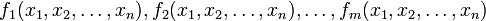$f_1(x_1,x_2,\dots,x_n), f_2(x_1,x_2,\dots,x_n),\dots,f_m(x_1,x_2,\dots,x_n)$. Suppose$(a_1,a_2,\dots,a_n)$ is a point in the domain of$f$ such that$f_i$ is differentiable at$(a_1,a_2,\dots,a_n)$ for$i \in \{ 1,2,\dots,m\}$. Then, the Jacobian matrix of$f$ at$(a_1,a_2,\dots,a_n)$ is a$m \times n$ matrix of numbers whose$i^{th}$ row is given by the gradient vector of$f_i$ at$(a_1,a_2,\dots,a_n)$.

Explicitly, in terms of rows, it looks like:$\begin{pmatrix} \nabla(f_1)(a_1,a_2,\dots,a_n) \\ \nabla(f_2)(a_1,a_2,\dots,a_n) \\ \cdot \\ \cdot \\ \cdot \\ \nabla(f_m)(a_1,a_2,\dots,a_n) \\\end{pmatrix}$

### Definition at a point in terms of partial derivatives

Suppose$f$ is a vector-valued function with$n$-dimensional inputs and$m$-dimensional outputs. Explicitly, suppose$f$ is a function with inputs$x_1,x_2,\dots,x_n$ and outputs$f_1(x_1,x_2,\dots,x_n), f_2(x_1,x_2,\dots,x_n),\dots,f_m(x_1,x_2,\dots,x_n)$. Suppose$(a_1,a_2,\dots,a_n)$ is a point in the domain of$f$ such that$f_i$ is differentiable at$(a_1,a_2,\dots,a_n)$ for$i \in \{ 1,2,\dots,m\}$. Then, the Jacobian matrix of$f$ at$(a_1,a_2,\dots,a_n)$ is a$m \times n$ matrix of numbers whose$(ij)^{th}$ entry is given by: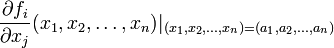$\frac{\partial f_i}{\partial x_j}(x_1,x_2,\dots,x_n)|_{(x_1,x_2,\dots,x_n) = (a_1,a_2,\dots,a_n)}$

Here's how the matrix looks: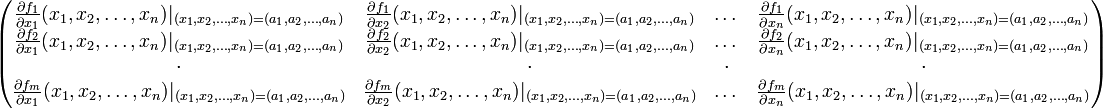$\begin{pmatrix} \frac{\partial f_1}{\partial x_1}(x_1,x_2,\dots,x_n)|_{(x_1,x_2,\dots,x_n) = (a_1,a_2,\dots,a_n)} & \frac{\partial f_1}{\partial x_2}(x_1,x_2,\dots,x_n)|_{(x_1,x_2,\dots,x_n) = (a_1,a_2,\dots,a_n)} & \dots & \frac{\partial f_1}{\partial x_n}(x_1,x_2,\dots,x_n)|_{(x_1,x_2,\dots,x_n) = (a_1,a_2,\dots,a_n)} \\ \frac{\partial f_2}{\partial x_1}(x_1,x_2,\dots,x_n)|_{(x_1,x_2,\dots,x_n) = (a_1,a_2,\dots,a_n)} & \frac{\partial f_2}{\partial x_2}(x_1,x_2,\dots,x_n)|_{(x_1,x_2,\dots,x_n) = (a_1,a_2,\dots,a_n)} & \dots & \frac{\partial f_2}{\partial x_n}(x_1,x_2,\dots,x_n)|_{(x_1,x_2,\dots,x_n) = (a_1,a_2,\dots,a_n)} \\ \cdot & \cdot & \cdot & \cdot \\ \frac{\partial f_m}{\partial x_1}(x_1,x_2,\dots,x_n)|_{(x_1,x_2,\dots,x_n) = (a_1,a_2,\dots,a_n)} & \frac{\partial f_m}{\partial x_2}(x_1,x_2,\dots,x_n)|_{(x_1,x_2,\dots,x_n) = (a_1,a_2,\dots,a_n)} & \dots & \frac{\partial f_m}{\partial x_n}(x_1,x_2,\dots,x_n)|_{(x_1,x_2,\dots,x_n) = (a_1,a_2,\dots,a_n)} \\\end{pmatrix}$

Note that for this definition to be correct, it is still necessary that the gradient vectors exist. If the gradient vectors do not exist but the partial derivatives do, a matrix can still be constructed using this recipe but it may not satisfy the nice behavior that the Jacobian matrix does.

## Definition as a function

### Definition in terms of gradient vectors as row vectors

Suppose$f$ is a vector-valued function with$n$-dimensional inputs and$m$-dimensional outputs. Explicitly, suppose$f$ is a function with inputs$x_1,x_2,\dots,x_n$ and outputs$f_1(x_1,x_2,\dots,x_n), f_2(x_1,x_2,\dots,x_n),\dots,f_m(x_1,x_2,\dots,x_n)$. Then, the Jacobian matrix of$f$is a$m \times n$ matrix of functions whose$i^{th}$ row is given by the gradient vector of$f_i$. Explicitly, it looks like this: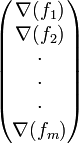$\begin{pmatrix} \nabla(f_1) \\ \nabla(f_2)\\ \cdot \\ \cdot \\ \cdot \\ \nabla(f_m) \\\end{pmatrix}$

Note that the domain of this function is the set of points at which all the$f_i$s individually are differentiable.

### Definition in terms of partial derivatives

Suppose$f$ is a vector-valued function with$n$-dimensional inputs and$m$-dimensional outputs. Explicitly, suppose$f$ is a function with inputs$x_1,x_2,\dots,x_n$ and outputs$f_1(x_1,x_2,\dots,x_n), f_2(x_1,x_2,\dots,x_n),\dots,f_m(x_1,x_2,\dots,x_n)$. Then, the Jacobian matrix of$f$ is a$m \times n$ matrix of functions whose$(ij)^{th}$ entry is given by: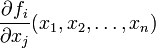$\frac{\partial f_i}{\partial x_j}(x_1,x_2,\dots,x_n)$

wherever all the$f_i$s individually are differentiable in the sense of the gradient vectors existing. Here's how the matrix looks:$\begin{pmatrix} \frac{\partial f_1}{\partial x_1} & \frac{\partial f_1}{\partial x_2}& \dots & \frac{\partial f_1}{\partial x_n}\\ \frac{\partial f_2}{\partial x_1} & \frac{\partial f_2}{\partial x_2} & \dots & \frac{\partial f_2}{\partial x_n}\\ \cdot & \cdot & \cdot & \cdot \\ \frac{\partial f_m}{\partial x_1} & \frac{\partial f_m}{\partial x_2} & \dots & \frac{\partial f_m}{\partial x_n}\\\end{pmatrix}$

If the gradient vectors do not exist but the partial derivatives do, a matrix can still be constructed using this recipe but it may not satisfy the nice behavior that the Jacobian matrix does.

## Particular cases

Case What happens in that case?$m = n = 1$$f$ is a real-valued function of one variable. The Jacobian matrix is a$1 \times 1$ matrix whose entry is the ordinary derivative.$n = 1$,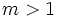$m > 1$$f$ is a vector-valued function of one variable. We can think of it as a parametric curve in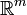$\R^m$. The Jacobian matrix is a$m \times 1$ matrix which, read as a column vector, is the parametric derivative of the vector-valued function.$m = 1$,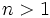$n > 1$$f$ is a real-valued function of multiple variables. The Jacobian matrix is a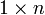$1 \times n$ matrix which, read as a row vector, is the gradient vector function.$f$ is a linear or affine map. The Jacobian matrix is the same as the matrix describing$f$ (or, if$f$ is affine, the matrix describing the linear part of$f$).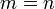$m = n$, and we are identifying the spaces of inputs and outputs of$f$. The Jacobian matrix can then be thought of as a linear self-map from the$n$-dimensional space to itself. In this context, we can consider the Jacobian determinant.